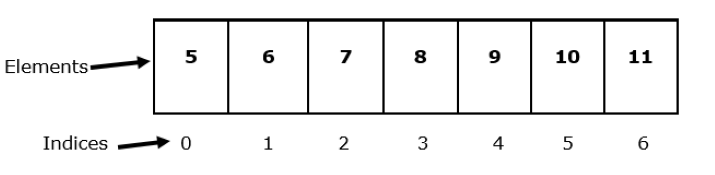# Java Program to Check Array Bounds while inputting Elements into the Array

Array is a linear data structure that is used to store group of elements with similar datatypes. It stores data in a sequential manner. Once we create an array we can’t change its size i.e. it is of fixed length.

This article will help you to understand the basic concept of array and array bound. Also, we will discuss java program to check array bounds while inputting elements into the array.

## Array and Array Bound

We can access elements of array by its index. Suppose we have an array of length N, thenWe can see in the above diagram that there are 7 elements in the array but index value is from 0 to 6 i.e. 0 to 7 - 1.

The range of the array is called as its bound. The range of the above array is from 0 to 6 therefore, we can also say that 0 to 6 is the bound of the given array. If we try to access the index value out of its range or negative index then, we will get ArrayIndexOutOfBoundsException. It is an error that occurs at runtime.

### Syntax to declare an array

Data_Type[] nameOfarray;
// declaration
Or,
Data_Type nameOfarray[];
// declaration
Or,
// declaration with size
Data_Type nameOfarray[] = new Data_Type[sizeofarray];
// declaration and initialization
Data_Type nameOfarray[] = {values separated with comma};


We can use any of the above syntaxes in our program.

Checking Array Bounds while inputting Elements into the Array

### Example 1

If we access the elements of array within its bound then we won’t get any error. Program will successfully executed.

public class Main {
public static void main(String []args) {
// declaration and initialization of array ‘item[]’ with size 5
String[] item = new String;
// 0 to 4 is the indices
item = "Rice";
item = "Milk";
item = "Butter";
item = "Peanut";
System.out.print(" Elements of the array item: " );
// loop will iterate till 4 and will print the elements of ‘item[]’
for(int i = 0; i <= 4; i++) {
System.out.print(item[i] + " ");
}
}
}


### Output

Elements of the array item: Rice Milk Bread Butter Peanut


### Example 2

Let’s try to print the value outside the range of the given array.

public class Tutorialspoint {
public static void main(String []args) {
String[] item = new String;
item = "Rice";
item = "Milk";
item = "Butter";
item = "Peanut";
// trying to run the for loop till index 5
for(int i = 0; i <= 5; i++) {
System.out.println(item[i]);
}
}
}


### Output

Rice
Milk
Butter
Peanut
Exception in thread "main" java.lang.ArrayIndexOutOfBoundsException: Index 5 out of bounds for length 5
at Tutorialspoint.main(Tutorialspoint.java:11)


As we have discussed earlier, if we try to access the index value of an array out of its range or negative index then, we will get ArrayIndexOutOfBoundsException.

In the above program, we have tried to execute the for loop till index 5 of array ‘item[]’ but its range is 0 to 4 only. Therefore, after printing the elements till 4 we got an error.

### Example 3

In this example, we try to handle the ArrayIndexOutOfBoundsException using try and catch block. We will check array bounds while inputting elements into the array from user.

import java.util.*;
public class Tutorialspoint {
public static void main(String []args) throws ArrayIndexOutOfBoundsException {
// Here ‘sc’ is the object of scanner class
Scanner sc = new Scanner(System.in);
System.out.print("Enter number of items: ");
int n = sc.nextInt();
// declaration and initialization of array ‘item[]’
String[] item = new String[n];
// try block to test the error
try {
// to take input from user
for(int i =0; i<= item.length; i++) {
item[i] = sc.nextLine();
}
}
// We will handle the exception in catch block
catch (ArrayIndexOutOfBoundsException exp) {
// Printing this message to let user know that array bound exceeded
System.out.println(
" Array Bounds Exceeded  \n Can't take more inputs ");
}
}
}


### Output

Enter number of items: 3


## Conclusion

In this article, we have learned about array and array bound. We have discussed why we will get an error if we try to access the elements of array outside its range and how we can handle this error using try and catch block.

Updated on: 02-May-2023

47 Views